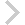FICO Xpress Optimization Examples Repository
 FICO Optimization Community FICO Xpress Optimization HomeNonlinear trimloss problem

Description
Nonlinear formulation of trim loss problem solved either via the Xpress Nonlinear solvers (trimminlp.mos) or configurable for using alternative NLP solvers (trimminnlp2.mos).

Further explanation of this example: This model is discussed in Section 13.3 of the book 'J. Kallrath: Business Optimization Using Mathematical Programming - An Introduction with Case Studies and Solutions in Various Algebraic Modeling Languages' (2nd edition, Springer, Cham, 2021, DOI 10.1007/978-3-030-73237-0).

Source Files
By clicking on a file name, a preview is opened at the bottom of this page.

trimminlp.mos

(!*********************************************************************
Mosel Example Problems
======================

file trimminlp.mos

Nonlinear formulation of trim loss problem

Example described in section 13.3 of
J. Kallrath: Business Optimization Using Mathematical Programming -
An Introduction with Case Studies and Solutions in Various Algebraic
Modeling Languages. 2nd edition, Springer Nature, Cham, 2021

author: S. Heipcke, October 2020

(c) Copyright 2020 Fair Isaac Corporation

you may not use this file except in compliance with the License.
You may obtain a copy of the License at

Unless required by applicable law or agreed to in writing, software
WITHOUT WARRANTIES OR CONDITIONS OF ANY KIND, either express or implied.
See the License for the specific language governing permissions and

*********************************************************************!)

model 'trimminlp'
uses "mmxnlp", "mmsystem"

!******** Input data ********
declarations
RPATT=1..6                    ! Set of cutting patterns
RWDTH=1..6                    ! Set of order width types
WDTH: array(RWDTH) of real    ! Widths of orders
DEM: array(RWDTH) of real     ! Requirements
CP: array(RPATT) of real      ! Pattern cost
CR: array(RPATT) of real      ! Roll cost
WMR: integer                  ! Width of master roll
FWIDTH: integer               ! Min fill width
KNIFES: integer               ! Max number of knifes available
MAXW: array(RWDTH) of real    ! Bound on the number of width in a pattern
end-declarations

WMR:= 2360; FWIDTH:= 2225; KNIFES:=12
WDTH::[300,280,265,240,225,208]
DEM::[6,6,9,9,12,15]
forall(p in RPATT) CP(p):=1
forall(p in RPATT) CR(p):=2

!******** Problem formulation ********
declarations
alpha: array(RWDTH,RPATT) of mpvar  ! Number of width i per pattern p
muse: array(RPATT) of mpvar         ! How oftern pattern is used
delta: array(RPATT) of mpvar        ! Indicate whether pattern is active
Numpat: linctr                      ! Objective: number of patterns used
end-declarations

! Upper bound on the number of width in a pattern
forall(i in RWDTH) MAXW(i):= minlist(floor(WMR/WDTH(i)),DEM(i))
forall(i in RWDTH,p in RPATT) do
alpha(i,p) is_integer
alpha(i,p) <= MAXW(i)
end-do

! Upper bound on pattern multiplicity
MAXDEM:= max(i in RWDTH) DEM(i)
forall(p in RPATT) do
muse(p) is_integer
muse(p) <= MAXDEM
end-do

forall(p in RPATT) delta(p) is_binary

! Objective function: weighted number of patterns + number of rolls used
Numpat:= sum(p in RPATT) (CP(p)*delta(p) + CR(p)*muse(p))

! Fulfill demand exactly (nonlinear, nonconvex constraint)
forall(i in RWDTH) Demand(i):= sum(p in RPATT) alpha(i,p)*muse(p) = DEM(i)

! Fit into the width of the master roll
forall(p in RPATT) MRwidth(p):= sum(i in RWDTH) WDTH(i)*alpha(i,p) <= WMR

! Observe the minimal width to be filled
forall(p in RPATT)
MinFill(p):= sum(i in RWDTH) WDTH(i)*alpha(i,p) >= FWIDTH*delta(p)

! Limit the maximal available number of knifes
forall(p in RPATT) Knifes(p):= sum(i in RWDTH) alpha(i,p) <= KNIFES

! Whether pattern p is used, that is, delta(p) = 1, else 0
forall(p in RPATT) PatternUse(p):= muse(p) <= MAXDEM*delta(p)

! Symmetry breaking: activate pattern p only when pattern p is active
forall(p in RPATT | p>RPATT.first) Symmetry1(p):= delta(p) <= delta(p-1)

! Symmetry breaking: order the patterns with descending multiplicity
forall(p in RPATT | p>RPATT.first) Symmetry2(p):= muse(p) <= muse(p-1)

! Linking alpha and delta variables
forall(i in RWDTH,p in RPATT) Cut1(i,p):= alpha(i,p) <= MAXW(i)*delta(p)

! Pattern needs to have a positive multiplicity to be used at all
forall(p in RPATT) Cut2(p):= delta(p) <= muse(p)

!******** Minimize the number of patterns ********

! Configuration of the solver
setparam("xnlp_verbose", true)

! Solve the problem
minimize(Numpat)
if getprobstat<>XPRS_OPT and getprobstat<>XPRS_UNF then
writeln("No solution available. Solver status: ", getparam("xnlp_status"))
else
writeln("Solution: ", getobjval,
". Number of patterns=", getsol(sum(p in RPATT) delta(p)),
". Number of rolls=", sum(p in RPATT) muse(p).sol )
write("          Width ")
forall(i in RWDTH) write(strfmt(WDTH(i),4))
writeln(" | Filled width")
write("       (Demand)")
forall(i in RWDTH) write(strfmt(DEM(i),4))
writeln("\n", "-"*60)
forall(p in RPATT | muse(p).sol>0) do
write("Pattern ", p, " x ", strfmt(muse(p).sol,2), ":")
forall(i in RWDTH) write(strfmt(alpha(i,p).sol,4))
writeln("  |   ",sum(i in RWDTH) WDTH(i)*alpha(i,p).sol)
end-do
end-if

end-model